Get instant live expert help with Excel or Google Sheets“My Excelchat expert helped me in less than 20 minutes, saving me what would have been 5 hours of work!”

#### Post your problem and you’ll get expert help in seconds.

Your message must be at least 40 characters
Our professional experts are available now. Your privacy is guaranteed.

# How to sum up if greater than or less than 0 – Excelchat

We can sum a data set with a specified criterion using the SUMIF function. The criteria could be for values to sum if greater than or sum if less than a number. This tutorial would teach us how to sum values if they are greater than or less than a specified value.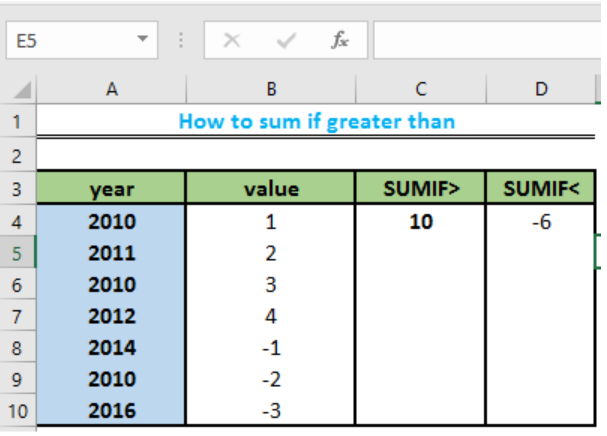Figure 1: SUMIF greater than or less than 0

## Setting up the Data

• We will prepare a table of data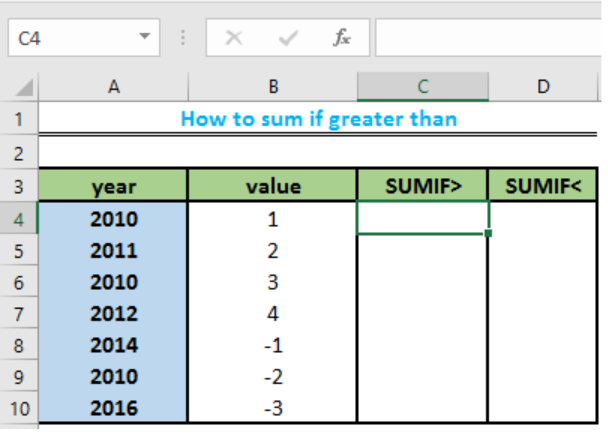Figure 2: Table of Data

• We will now click on Cell C4 to type the formula =SUMIF(B4:B10, “>0”). 0 specifies that all numbers greater than 0 within the range B4:B10 is summed.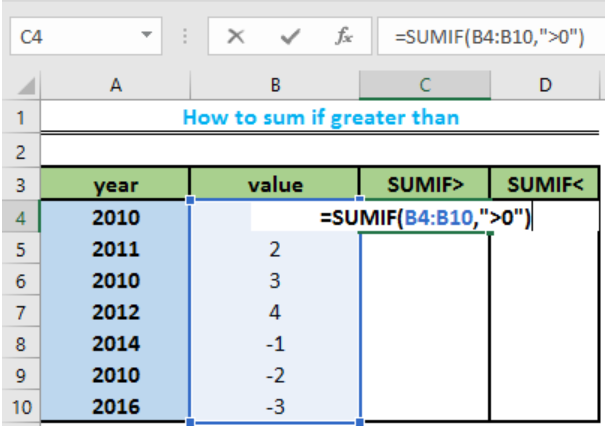Figure 3a: Formula for SUMIF values greater than 0

• We will now press the enter key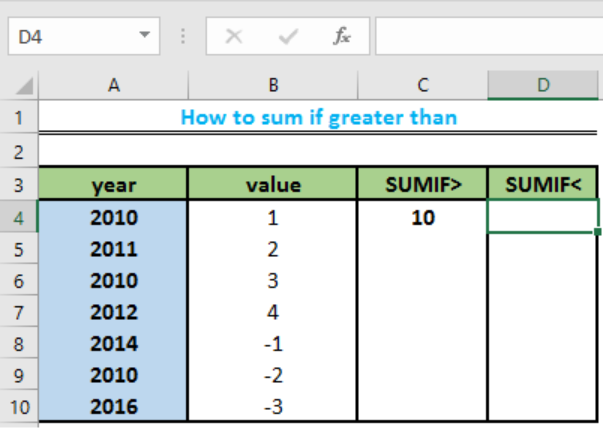Figure 3b: Answer for the SUMIF values greater than 0

• Also, we will create a column for SUMIF less than 0 by typing this formula =SUMIF(B4:B10,”<0″) into Cell D4 This will sum the values less than 0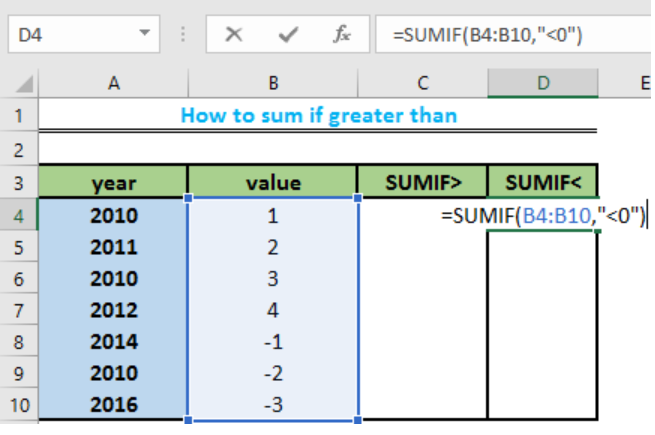Figure 4a: Formula for SUMIF values less than 0

• We will now press the enter key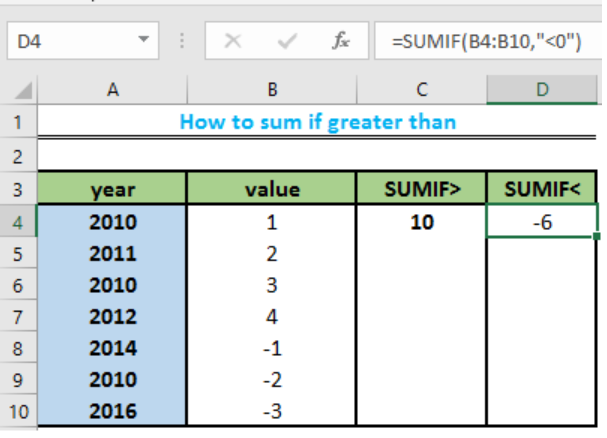Figure 4b: Answer for SUMIF values less than 0

## Instant Connection to an Expert through our Excelchat Service

Most of the time, the problem you will need to solve will be more complex than a simple application of a formula or function. If you want to save hours of research and frustration, try our live Excelchat service! Our Excel Experts are available 24/7 to answer any Excel question you may have. We guarantee a connection within 30 seconds and a customized solution within 20 minutes.